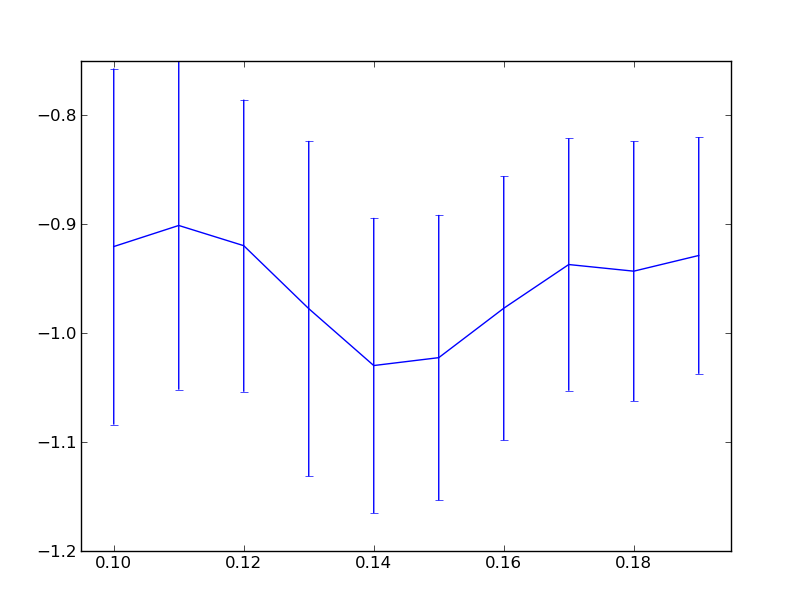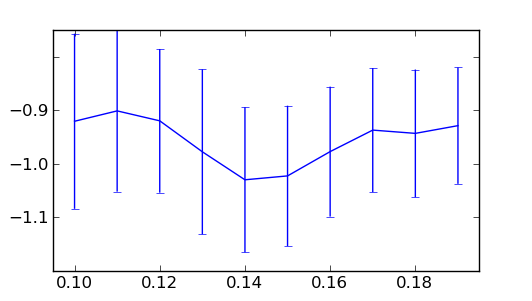# hide labels

Dear all,

I’m producing a single figure with subplots arrange in a single columns. They all share the same x range but the y variable change from subplot to subplot

In order have a nicer figure I hide the first and the last y label of each subplot in the following way

ytl = subpl.get_ymajorticklabels()
ytl.set_visible(False)
ytl[-1].set_visible(False)

It was well in most cases. But I’ve noticed that in some plot the first and/or the last label remains.
In this cases, if I “print ytl”, it writes “”, where “n” is larger by 1 or 2 than the number of labels shown before I make them invisible.

So I end up having some label (nearly) exactly on the upper and/or bottom range of the plot.

Is there a way to force the axis to return exactly the number of labels shown in the plot?

Francesco

Dear all again,

I've tried to play with it again, but I couldn't find a solution for
the problem.
For clarity I report an example of what each of the subplots looks like:

import numpy as np
import matplotlib.pyplot as plt
mean = np.array([-0.9206394, -0.90127456, -0.91983625, -0.97765539, -1.02991184, -1.02267017, -0.97730167, -0.93715172, -0.94324653, -0.92884379])
stddev = np.array([0.16351397, 0.15075966, 0.13413909, 0.15404823, 0.13559582, 0.13109754, 0.12128598, 0.11589682, 0.11921571, 0.10866761])

ax.errorbar(np.arange(10,20)/100., mean, yerr=stddev)

ax.set_xlim([0.095, 0.195])
lab = ax.get_ymajorticklabels()

print lab
for i in lab:
print i

plt.show()

as output of this script I get

<a list of 7 Text yticklabel objects>
Text(0,0,'')
Text(0,0,'')
Text(0,0,'')
Text(0,0,'')
Text(0,0,'')
Text(0,0,'')
Text(0,0,'')

In the plot instead I only have only 5 y tick labels, as visible from
the attachment. In this case if I set the first and the last label
invisible, nothing changes, and if I have other plots under this the

I hope to have been clearer than in my previous mail.
Any suggestion on how to fix or find a workaround in order to get the
same number of tick labels as the ones actually plotted.

Fra

2011/2/1 Francesco Montesano <franz.bergesund@...982...>:···

Dear all,

I'm producing a single figure with subplots arrange in a single columns.
They all share the same x range but the y variable change from subplot to
subplot

In order have a nicer figure I hide the first and the last y label of each
subplot in the following way

ytl = subpl.get_ymajorticklabels()
ytl.set_visible(False)
ytl[-1].set_visible(False)

It was well in most cases. But I've noticed that in some plot the first
and/or the last label remains.
In this cases, if I "print ytl", it writes "<a list of n Text yticklabel
>", where "n" is larger by 1 or 2 than the number of labels shown
before I make them invisible.
So I end up having some label (nearly) exactly on the upper and/or bottom
range of the plot.

Is there a way to force the axis to return exactly the number of labels
shown in the plot?

Francesco

--
personals: montyfra@...174..., monte_fra@...32... (messenger),
franz.bergesund@...3264...
work: montefra@...2911...

Francesco Montesano, on 2011-02-04 17:01, wrote:

Dear all again,

I've tried to play with it again, but I couldn't find a
solution for the problem. For clarity I report an example of
what each of the subplots looks like:

Hi Francesco,

thanks for the clarification, here are two ways to get the look
going on before. (The resulting figure is attached, just in case).

import numpy as np
import matplotlib.pyplot as plt
mean=np.array([-0.9206394, -0.90127456, -0.91983625, -0.97765539, -1.02991184,
-1.02267017, -0.97730167, -0.93715172, -0.94324653, -0.92884379])
stddev= np.array([0.16351397,0.15075966,0.13413909,0.15404823,0.13559582, 0.13109754,0.12128598,0.11589682,0.11921571,0.10866761])

ax.errorbar(np.arange(10,20)/100., mean, yerr=stddev)

ax.set_xlim([0.095, 0.195])

lab = ax.get_ymajorticklabels()
plt.draw() # ticks only get text assigned during a call to draw
print lab
for i in lab:
print i # note that \u2212 is a unicode minus sign

# this work for the first draw - relies on l.get_text() returning
# nothing for labels which aren't used/drawn - which isn't the
# case in general after panning and zooming interactively
shown_lab = [l for l in lab if l.get_text()]
shown_lab.set_visible(False)
shown_lab[-1].set_visible(False)

## alternative solution without extra draw(). more robust, can be
## used even after initial draw.
#ymin,ymax = ax.get_ylim()
#tl = ax.yaxis.get_majorticklocs()
#lab[(tl<ymin).sum()].set_visible(False)
#lab[-(tl>ymax).sum()-1].set_visible(False)

## hybrid of the two.
#ymin,ymax = ax.get_ylim()
#tl = ax.yaxis.get_majorticklocs()
#shown_lab = [l for l,t in zip(lab,tl) if t>ymin and t<ymax)
#shown_lab.set_visible(False)
#shown_lab[-1].set_visible(False)

plt.show()

best,···

--
Paul Ivanov
314 address only used for lists, off-list direct email at:
http://pirsquared.org | GPG/PGP key id: 0x0F3E28F7

For an interactive use, you may use callbacks to update the visibility
of ticks automatically.
Regards,

-JJ

import matplotlib.transforms as mtransforms

def update_yticks(ax):

axis = ax.yaxis
interval = axis.get_view_interval()

# get visible ticks
myticks = [t for t in axis.iter_ticks() \
if mtransforms.interval_contains(interval, t)]

# make all ticks visible again
for mytick in myticks: mytick.label1.set_visible(True)

# make first tick invisible
myticks.label1.set_visible(False)

# make last tick invisible
myticks[-1].label1.set_visible(False)

import matplotlib.pyplot as plt

ax = plt.subplot(111)
update_yticks(ax)
cid = ax.callbacks.connect('ylim_changed', update_yticks)

···

On Sun, Feb 6, 2011 at 5:17 PM, Paul Ivanov <pivanov314@...287...> wrote:

Francesco Montesano, on 2011-02-04 17:01, wrote:

Dear all again,

I've tried to play with it again, but I couldn't find a
solution for the problem. For clarity I report an example of
what each of the subplots looks like:

Hi Francesco,

thanks for the clarification, here are two ways to get the look
going on before. (The resulting figure is attached, just in case).

import numpy as np
import matplotlib.pyplot as plt
mean=np.array([-0.9206394, -0.90127456, -0.91983625, -0.97765539, -1.02991184,
-1.02267017, -0.97730167, -0.93715172, -0.94324653, -0.92884379])
stddev= np.array([0.16351397,0.15075966,0.13413909,0.15404823,0.13559582, 0.13109754,0.12128598,0.11589682,0.11921571,0.10866761])

ax.errorbar(np.arange(10,20)/100., mean, yerr=stddev)

ax.set_xlim([0.095, 0.195])

lab = ax.get_ymajorticklabels()
plt.draw() # ticks only get text assigned during a call to draw
print lab
for i in lab:
print i # note that \u2212 is a unicode minus sign

# this work for the first draw - relies on l.get_text() returning
# nothing for labels which aren't used/drawn - which isn't the
# case in general after panning and zooming interactively
shown_lab = [l for l in lab if l.get_text()]
shown_lab.set_visible(False)
shown_lab[-1].set_visible(False)

## alternative solution without extra draw(). more robust, can be
## used even after initial draw.
#ymin,ymax = ax.get_ylim()
#tl = ax.yaxis.get_majorticklocs()
#lab[(tl<ymin).sum()].set_visible(False)
#lab[-(tl>ymax).sum()-1].set_visible(False)

## hybrid of the two.
#ymin,ymax = ax.get_ylim()
#tl = ax.yaxis.get_majorticklocs()
#shown_lab = [l for l,t in zip(lab,tl) if t>ymin and t<ymax)
#shown_lab.set_visible(False)
#shown_lab[-1].set_visible(False)

plt.show()

best,
--
Paul Ivanov
314 address only used for lists, off-list direct email at:
http://pirsquared.org | GPG/PGP key id: 0x0F3E28F7

-----BEGIN PGP SIGNATURE-----
Version: GnuPG v1.4.10 (GNU/Linux)

iEYEARECAAYFAk1OWQMACgkQe+cmRQ8+KPekfgCfcY+R1vb2i/l/AoVsFZwsyqCC
ihoAn1uni4kEu4Kq+B0IdCu26Kw1aA9Q
=B6ZO
-----END PGP SIGNATURE-----

------------------------------------------------------------------------------
The modern datacenter depends on network connectivity to access resources
and provide services. The best practices for maximizing a physical server's
connectivity to a physical network are well understood - see how these
rules translate into the virtual world?
http://p.sf.net/sfu/oracle-sfdevnlfb
_______________________________________________
Matplotlib-users mailing list
Matplotlib-users@lists.sourceforge.net
https://lists.sourceforge.net/lists/listinfo/matplotlib-users